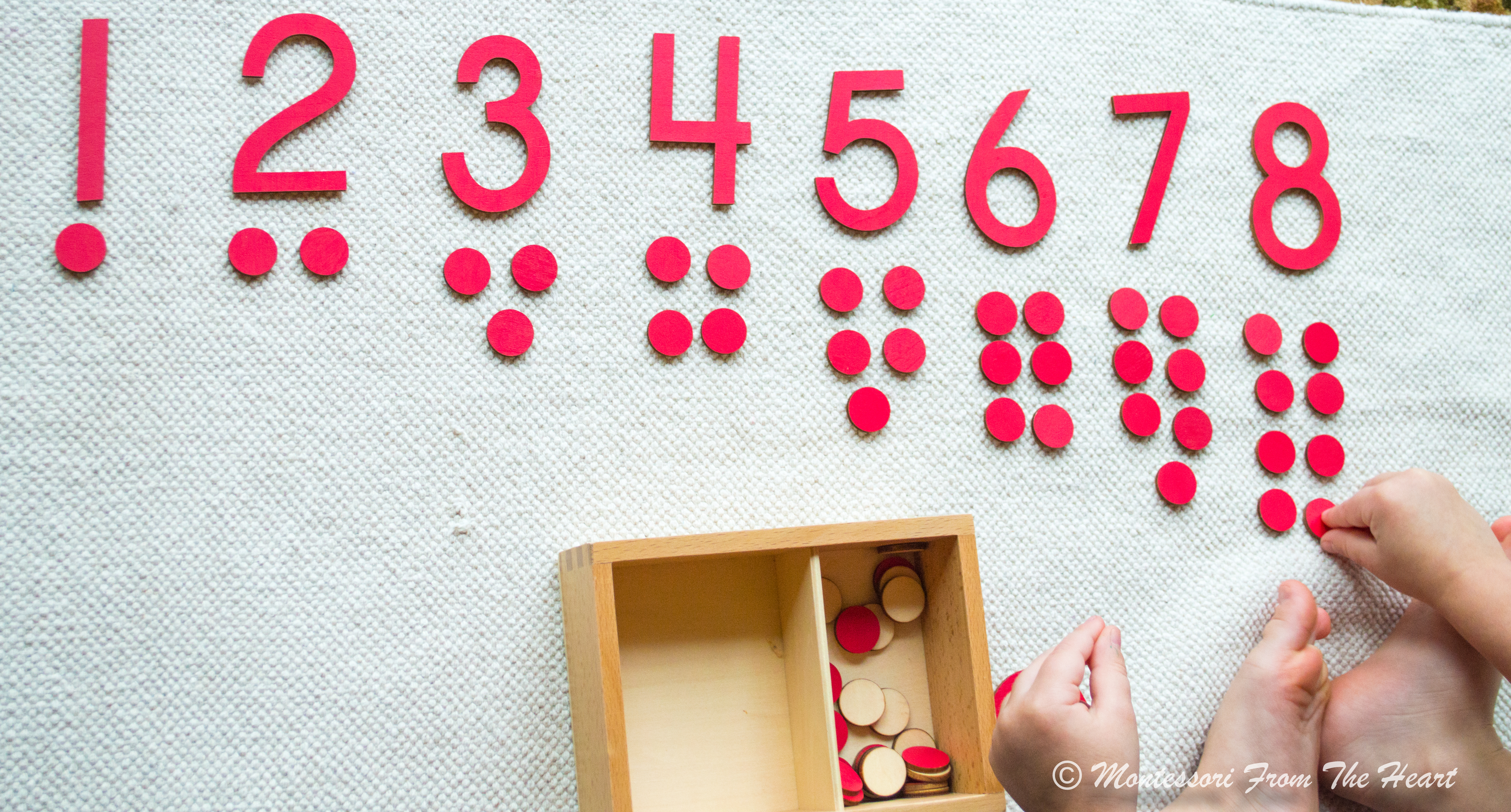Montessori Odd and Even Math lesson (also called cards and counters) concretely and visually teaches a child what odd and even numbers are. You can use a traditional Montessori Numerals and Counters (buy here) or you can make it yourself: you would need numbers 1-10 and 55 counters (you can use marbles, wooden dots, or holiday-inspired counters.Presentation: place numbers in random order and ask your child to set numbers one through ten horizontally at the top of the rug. You want to make sure that your child knows numbers one through ten before introducing the concept of odd/even.

Position counters in pairs. If the number is odd, one counter should be positioned separately.

By positioning counters in pairs, you offer a child a concrete visual representation to determine which number has a counter without a pair/on its own: meaning that the number is odd. As opposed to, when all counters have a complete set of pairs - meaning that the number is evenI suggest introducing this material not earlier than three years of age since although concrete this activity still introduces a very abstract concept of odd vs even. Adrian just turned 38 months, and this is still confusing to him, even with all the visual counters, so I will wait a month and re-introduce it later.

See here "❤️Odd & Even (Montessori 🔢 Math 101 🎥 Series 🎇)" a video-post where Adrian summarizes how to determine which number is odd and which one is even.

Also, see here "🍁Fall-Inspired Odd and Even (Numbers & Counters)."

p.s. Montessori math curriculum is generally introduced in the following order: (1) Number Rods (the first material to introduce to a child at about two years of age), then (2) Sandpaper Numbers, (3) Spindle Box, (4) Numbers Memory Game, followed by Odd and Even activity (as shown above).